Next: Hard Sphere Scattering Up: Scattering Theory Previous: Partial Waves

# Determination of Phase-Shifts

Let us now consider how the phase-shiftsin Eq. (1306) can be evaluated. Consider a spherically symmetric potential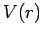which vanishes for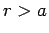, whereis termed the range of the potential. In the region, the wavefunctionsatisfies the free-space Schrödinger equation (1285). The most general solution which is consistent with no incoming spherical-waves is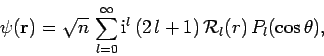(1309)

where(1310)

Note thatfunctions are allowed to appear in the above expression, because its region of validity does not include the origin (where). The logarithmic derivative of theth radial wavefunction,, just outside the range of the potential is given by(1311)

wheredenotes, etc. The above equation can be inverted to give(1312)

Thus, the problem of determining the phase-shiftis equivalent to that of obtaining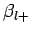.

The most general solution to Schrödinger's equation inside the range of the potential (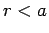) which does not depend on the azimuthal angle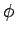is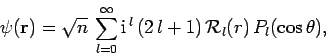(1313)

where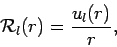(1314)

and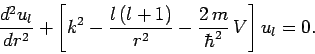(1315)

The boundary condition(1316)

ensures that the radial wavefunction is well-behaved at the origin. We can launch a well-behaved solution of the above equation from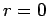, integrate out to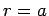, and form the logarithmic derivative(1317)

Sinceand its first derivatives are necessarily continuous for physically acceptible wavefunctions, it follows that(1318)

The phase-shiftis then obtainable from Eq. (1312).Next: Hard Sphere Scattering Up: Scattering Theory Previous: Partial Waves
Richard Fitzpatrick 2010-07-20